#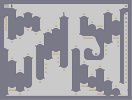Hover over the thumbnail for a full-size version.

Author kirbs action author:kirbs playable unrated 2005-12-27 2 more votes required for a rating. \$Gold is your friend#kirbs#none#01190000000:1111111111=01111111<00000;11111<000:111111=00000:11111=00000;111<000000000;111<0000:111=000000000:111=000;11<0000000;111111<0000:11=0000000:111111=00000;11<0000000000000000000:11=000;1111111<00000000000000:1111111=00000000000;11111<0000000000000000:11111=000000000000000;1111<00000000000000000:1111=00000000000000;11111111111<0000000000:11111111111=00000000000000000000000000000000000000000;111111111<000000000000:111111111=0000;111<0000000;111<000000:1111000;1<0:111=00000000;11000:110000;1<0000000:118000610000:1=00000000;11LH07100;1111<0000000:111111=00:1111=000;111111<000000000;11<00:111111=000000000:11=0000;11<0000000000000;<0000:11=0000000000000:=0;11111111111111111<00001900000000000000000000|5^384,84!12^576,336!12^96,444!12^144,444!12^48,372!12^264,276!12^312,204!12^360,180!12^528,156!12^576,180!12^672,132!12^480,396!12^528,468!12^576,468!12^624,492!12^672,540!12^48,516!12^96,516!12^144,516!12^312,300!12^360,300!12^264,348!12^480,492!12^528,492!12^576,516!12^624,540!12^672,564!12^624,180!12^624,252!12^672,204!12^720,204!12^720,132!0^756,492!0^756,468!0^756,444!0^756,420!0^756,396!0^756,372!0^756,348!0^756,324!0^756,300!0^756,276!0^756,252!0^756,228!0^756,204!0^756,180!0^756,156!12^360,108!12^408,108!12^480,108!12^528,108!12^360,396!12^312,348!12^264,204!12^312,180!12^216,276!12^192,348!12^144,348!12^96,372!12^48,300!12^264,468!12^48,564!12^96,540!12^144,540!12^432,540!12^480,540!12^528,516!12^576,540!12^624,564!12^432,300!12^528,396!12^576,420!12^624,420!12^672,492!12^720,564!12^624,84!12^672,84!12^552,300!12^720,60!11^36,36,576,324!12^96,60!12^96,108!12^144,108!12^144,84!12^192,84!12^192,108!12^240,108!12^240,180!12^192,180!12^192,156!12^144,156!12^96,204!12^96,228!12^48,228!12^480,204!12^672,252!0^36,108!0^36,120!0^36,132!0^36,156!0^36,144!0^36,168!0^36,180!12^768,540!0^492,516!0^516,516!0^504,516!0^552,540!0^612,564!0^588,564!0^564,540!0^540,540!0^600,564!0^60,540!0^84,540!0^72,540!0^276,384!0^276,408!0^276,432!0^324,324!0^336,324!0^348,324!0^348,348!0^348,372!12^756,84!12^756,108!12^756,132!0^516,228!0^528,228!0^552,228!0^540,228!0^504,228!0^636,276!0^636,300!0^636,324!0^684,228!0^696,228!0^708,228!0^708,252!0^708,276!0^120,420!0^588,156!0^600,156!0^612,156!0^552,132!0^696,108!9^756,36,0,0,2,1,1,0,0!0^72,348!0^168,324!0^240,252!0^288,180!0^336,156!0^504,84!0^648,60!0^456,276!0^504,372!0^552,444!0^600,396!0^648,468!0^696,516!12^720,492!0^240,492!0^384,420!0^456,564!0^216,204!0^120,228!0^72,252!0^168,540!0^120,564!0^168,180# Try to collect all gold.

## Other maps by this author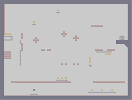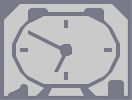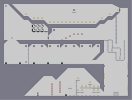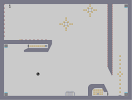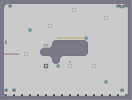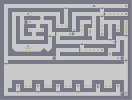Balloon Fighter Alarm Clock Exile Precision Cloud in the sky You know it's a door

Pages: (0)

### C+Z

Yeah, I know what they are. I'll edit this map later/soon/some time.

### Ugh

Another word of advice, those mines on the circular shapes look much too out of place. Try using the or snap to place them ON the circles.
If you don't know what the and snaps are, go into debug editor mode and press or in the item placer mode.